NZ Geomechanics News

# Converting Hoek-Brown strength envelope to Mohr Coulomb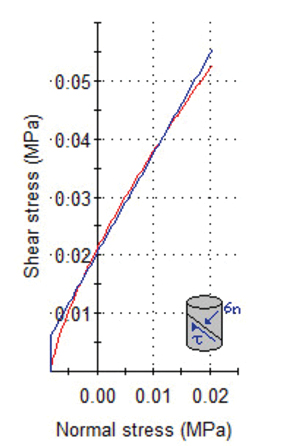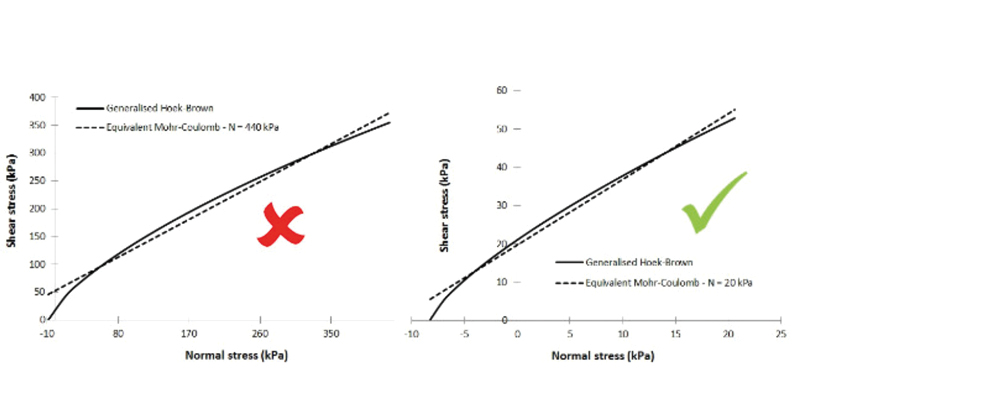The Generalised Hoek-Brown (GHB) criterion is likely to be, in one form or another, the most widely used rock mass strength criterion in geotechnical engineering practice, despite its semi-empirical nature or any other limitation. Perhaps the main reason is that, if used correctly, it should generally provide a safe assessment of rock mass strength due to its inherent assumptions and limitations.

One of its main advantages is that it captures the intuitive non-linear strength of rock masses. Well, if both intact rock and discontinuities are non-linear, we could not expect anything different for the rock mass.

In the past, not many computer codes or analysis methods had the GHB criterion implemented. Therefore, the need to convert the non-linear GHB strength envelope to an equivalent linear Mohr-Coulomb (MC) was quite common. Nowadays, it is different and the majority of computer programs have the GHB criterion implemented to some extent. As a result, this would seem unnecessary today. In fact one could risk making the wrong conversion, and assuming that the GHB is more realistic, obtain an unsafe assessment.

For one reason or another, one could still need or want to obtain MC parameters, for example, simplicity, an analytical procedure only developed for MC or any other reason. There is no problem in doing so. However, due to the non-linearity it is important that the engineer select an appropriate stress range which is representative of the failure mechanism under assessment.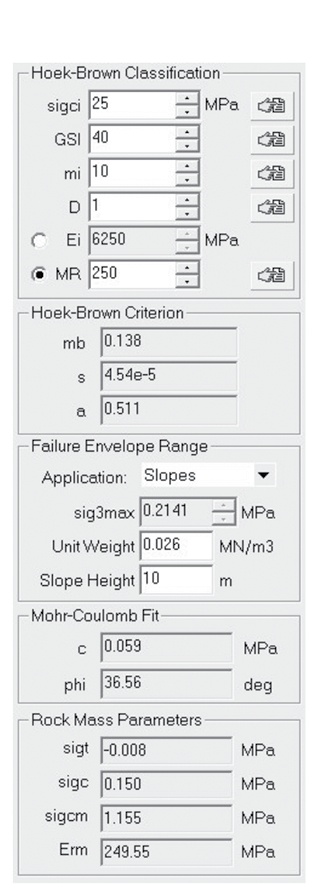Figure 1: Extract from RocLab input.

In making such a conversion, it is very common for geotechnical engineers to use the (now discontinued) program RocLab from Rocscience. The main two reasons perhaps are: (1) convenience due to its nice user-friendly interface, and (2) the research and theoretical background with involvement of Prof. Evert Hoek.
RocLab offers options to convert the GHB criterion to an MC for different applications: tunnels, slopes, general and a custom option. For example, in a slope “application”, RocLab only requests the rock unit weight and the slope height (obviously in addition to the GHB parameters) which confirms its convenience.
Figure 1 below depicts an example of a hypothetical 10m rock slope and rock strength. It presents the data entered in RocLab and the resulting MC fit with friction angle phi=37º and cohesion c = 59 kPa.
The above best-fit was performed by RocLab over a confining stress range of 0 to 214 kPa (i.e. sig3max of Figure 1). This confinement is equivalent to a normal stress along the failure plane of approximately 420 kPa. The best-fit depicted in Figure 2 below shows a good agreement except for normal stress below 80 kPa resulting in an expected overestimation of cohesion (always).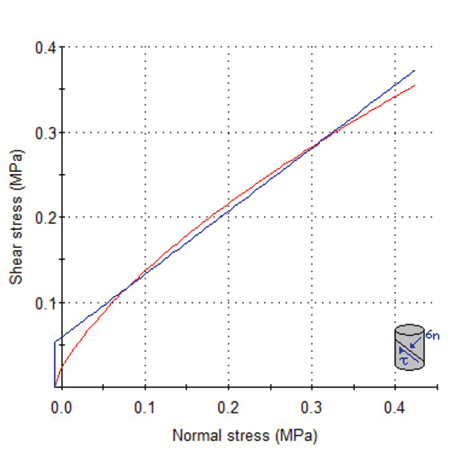Figure 2: Mohr-Coulomb fit.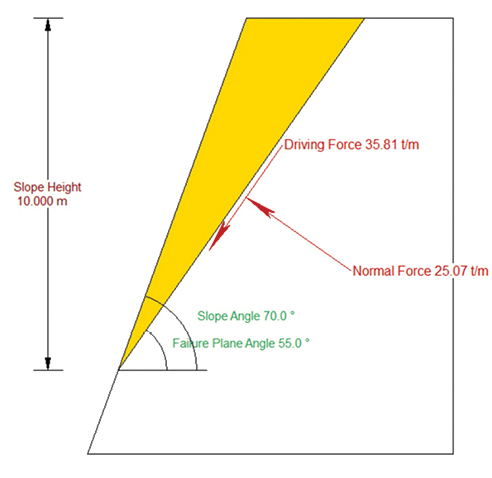Figure 3: Rock slope example.

The question that I now post, and would like you to answer in your mind before reading what follows is: As we selected “slope application” in RocLab, would you expect the above MC fit to be valid for any rock slope assessments where the height is 10m and the rock strength is reasonably represented by that given in Figure 1?
OK, let’s look at a hypothetical example. For instance, let’s assume a hypothetical 10m high slope with a flat bench and batter angle of 70%. There are concerns about potential planar failure at 55º corresponding to the dip angle of one set of discontinuities. The discontinuities are not fully persistent (or have rock bridges), so that we are comfortable to adopt the GHB strength envelope of Fig.1 and plotted in Figure 2.

This problem could be easily calculated by hand but for convenience I will use RocPlane program also from Rocscience. The resulting wedge is depicted in Figure 3.

If we use the GHB criterion in RocPlane, we obtain a factor of safety FS=1.91 that, for comparison purposes, we will assume to be the “true” FS.

If we now adopt the RocLab best fit for a “10m high slope” application, with phi=37º and c=59kPa, we obtain a FS=2.3, i.e. worryingly 20% higher than the “true” value. Why?
Well, from Figure 3 above it is evident that such rock wedge near the free rock slope face could never be under the maximum confinement of 214 kPa assumed in RocLab (sig3max). Therefore, the maximum normal stress is not 420 kPa either. In fact, if we look at the deepest point of the failure plane below the slope crest, we observe that the maximum normal stress would be about 130 kPa (i.e. 5m of rock), at best, but varying to zero at both ends of the wedge. If we now change the RocLab from a “slope application” (although true) to a “custom” application, and request a best-fit from 0 to 130 kPa normal stress (about 125 kPa confinement) we now obtain phi=45º and c=33kPa, as shown in Figure 4 below.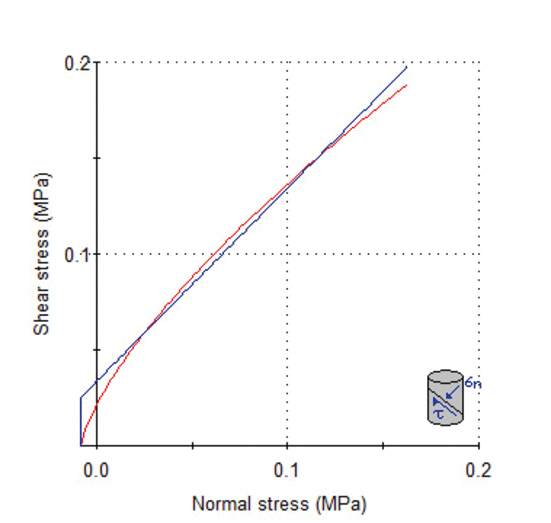Figure 4: Custom Mohr-Coulomb fit (130 kPa normal).

With the latter parameters (i.e. phi=45º and c=33kPa), the RocPlane factor of safety becomes FS=1.82. In other words, such stress range provides a slightly conservative assessment compared to the “true” value, despite the higher cohesion. This is caused by the underestimation of the strength for the average normal stress along the failure plane.

The interesting paper by Li et al (2008) in fact demonstrated that the RocLab equation for estimating Sig3max is quite inaccurate for steep slopes with batter angle greater than 45º (see Figure 5 below – dotted line).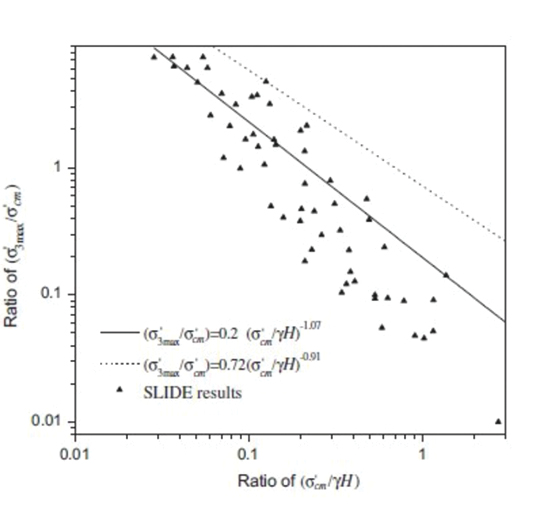Figure 5: Relationship for the calculation of Sig3max between equivalent Mohr–Coulomb and Hoek–Brown parameters for steep slopes – beta>45º (after Li et al. 2008).

If we use Li et al (2008) proposed equation (given in Figure 5 as solid line), the estimated sig3max is in fact 46.8 kPa which is equivalent to approximately 120 kPa normal stress along the failure plane, i.e. very close to the rough estimate above.

We could also estimate the average normal stress along the failure plane by taking the normal component of the self-weight, shown to be approximately 25t/m in Fig.3. Dividing 25t/m over the failure plane length (about 12m) we get an average normal stress of 20 kPa, i.e. well below the 420 kPa.Figure 6: Custom Mohr-Coulomb fit (20 kPa normal).

If we now request a best-fit from 0 to 20 kPa normal stress (basically only 3 kPa confinement) we obtain phi=60º and c=20kPa. Note in Figure 6 below that the cohesion now matches that of the GHB. With these parameters, RocPlane now gives a FS=1.89, practically the same as the “true” value which had also assumed an average normal stress.]

The above example illustrates the importance of adopting appropriate stress ranges for converting a GHB envelope to MC. Despite the convenience provided by RocLab and the false impression that a slope application would be appropriate in this case, it is important that we look at the particular failure mechanism under analysis. If one attempts to understand the source of the RocLab conversion for slopes, he/she would realize that is was developed for “Bishop’s circular failure analysis”, which is not the case above.

An appropriate stress range is equally important when deciding on lab testing where confinement or normal stress is applied.

In summary, it is possible to convert to a MC envelope with reasonable agreement to the GHB criterion and obtain negligible difference. However, it is also evident that adopting a non-linear strength may avoid significant work to adjust the stress range when several geometry cases or different mechanisms are under analysis. Therefore, it saves time/money and reduces the risks of one selecting an inappropriate stress range.

### REFERENCE

A.J. Li, R.S. Merifield and A.V. Lyamin (2008). Stability charts for rock slopes based on the Hoek–Brown failure criterion. International Journal of Rock Mechanics & Mining Sciences 45 (2008) 689–700.
The views and opinions expressed within this post are my own and do not represent the views or opinion of any third parties.Published
07/10/2020
Collection
Authors(s)
Compilation
N/A
Issue
99
Volume
N/A
Version
N/A
Location
N/A
Type
Tags
N/A
ISBN
ISSN
0111-6851